# JP2008256602A - Sectional image processing method, device, and program - Google Patents

## Info

Publication number
JP2008256602A
JP2008256602A JP2007100690A JP2007100690A JP2008256602A JP 2008256602 A JP2008256602 A JP 2008256602A JP 2007100690 A JP2007100690 A JP 2007100690A JP 2007100690 A JP2007100690 A JP 2007100690A JP 2008256602 A JP2008256602 A JP 2008256602A
Authority
JP
Japan
Prior art keywords
light
interference signal
tomographic image
interference
compensation
Prior art date
Legal status (The legal status is an assumption and is not a legal conclusion. Google has not performed a legal analysis and makes no representation as to the accuracy of the status listed.)
Withdrawn
Application number
JP2007100690A
Other languages
Japanese (ja)
Inventor
Kensuke Terakawa

Original Assignee
Fujifilm Corp

Priority date (The priority date is an assumption and is not a legal conclusion. Google has not performed a legal analysis and makes no representation as to the accuracy of the date listed.)
Filing date
Publication date
Application filed by Fujifilm Corp, 富士フイルム株式会社 filed Critical Fujifilm Corp
Priority to JP2007100690A priority Critical patent/JP2008256602A/en
Publication of JP2008256602A publication Critical patent/JP2008256602A/en
Application status is Withdrawn legal-status Critical

• 238000003672 processing method Methods 0 title 1
• 230000003287 optical Effects 0 abstract 2
• 238000003325 tomography Methods 0 abstract 2
• 230000014509 gene expression Effects 0 abstract 1

## Images

•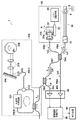•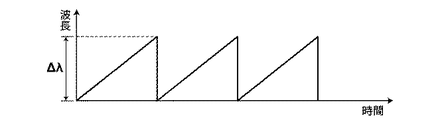••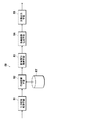•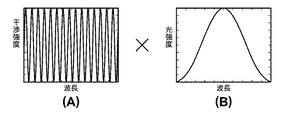••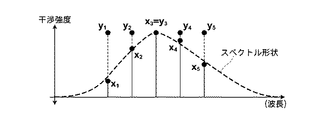•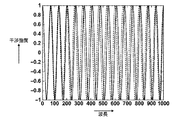•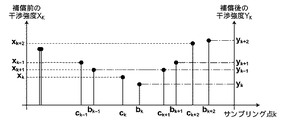•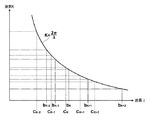••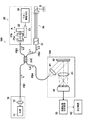## Abstract

<P>PROBLEM TO BE SOLVED: To efficiently create a sectional image by optical tomography measurement. <P>SOLUTION: When an interference signal IS is acquired by OCT (Optical Coherence Tomography) measurement, compensation of the amplitude direction of the interference signal IS and compensation of the phase direction are simultaneously performed using a compensation table RT shown in expression (1). Next, a sectional information acquiring means 53 acquires sectional information r(z) from the interference signal IS10 after the compensation, and a sectional image creating means 54 creates a sectional image P using the sectional information r(z). Then, sectional image P is displayed on a display device 60 by an image outputting means 55. <P>COPYRIGHT: (C)2009,JPO&INPIT

## Description

The present invention relates to a tomographic image processing method, apparatus, and program for generating an optical tomographic image by OCT (Optical Coherence Tomography) measurement.

Conventionally, it has been proposed to use an optical tomographic image acquisition apparatus using OCT measurement when acquiring an optical tomographic image of a living tissue. For example, in addition to acquiring tomographic images of the fundus, anterior eye, and skin, various observations such as observation of the arterial blood vessel wall using an optical probe, observation of the digestive tract that inserts the optical probe from the forceps channel of the endoscope Applied to the site. In this optical tomographic image acquisition apparatus, after the low-coherent light emitted from the light source is divided into measurement light and reference light, reflected light from the measurement object when the measurement light is irradiated to the measurement object, or backscattering The light and the reference light are combined, and an optical tomographic image is acquired based on the intensity of the interference light between the reflected light and the reference light. Hereinafter, the reflected light and the backscattered light from the measurement object are collectively referred to as reflected light.

The OCT measurement is roughly divided into two types: TD-OCT (Time domain OCT) measurement and FD (Fourier Domain) -OCT measurement. In TD-OCT (Time domain OCT) measurement, reflected light corresponding to a position in the depth direction of a measurement target (hereinafter referred to as a depth position) is measured by measuring the intensity of interference light while changing the optical path length of the reference light. This is a method for obtaining an intensity distribution.

On the other hand, the FD (Fourier Domain) -OCT measurement measures the interference light intensity for each spectral component of the light without changing the optical path lengths of the reference light and the signal light, and uses the obtained spectral interference intensity signal as a computer. In this method, the reflected light intensity distribution corresponding to the depth position is obtained by performing frequency analysis represented by Fourier transform. In recent years, it has attracted attention as a technique that enables high-speed measurement by eliminating the need for mechanical scanning existing in TD-OCT. Typical examples of the apparatus configuration for performing FD (Fourier Domain) -OCT measurement include an SD-OCT (Spectral Domain OCT) apparatus and an SS-OCT (Septt source OCT) (for example, Patent Document 1, Non-patent document 1).

The interference signal acquired by the FD-OCT measurement described above is detected in a state where the spectral intensity component of the light emitted from the light source unit is multiplied by the interference component. Therefore, since only the interference component is extracted from the interference signal, it is necessary to remove the spectrum component from the interference signal.

In the case of SS-OCT measurement, the interference signal detects the interference intensity with respect to time change (wavelength change) as an interference signal. On the other hand, in order to acquire tomographic information by performing frequency analysis such as Fourier transform on the interference signal, an interference signal having an interference intensity with respect to a wave number change is required, and it is necessary to perform signal conversion as shown in Patent Document 1. .
US 5956355 specification JP 2005-283155 A

Thus, in order to acquire tomographic information (reflectance) from the detected interference signal, it is necessary to perform preprocessing in the amplitude direction of the interference signal and preprocessing in the phase direction of the interference signal. However, since the preprocessing in the amplitude direction and the preprocessing in the phase direction as described above are performed separately for the interference signal, it takes time to preprocess the interference signal, and acquisition of tomographic information and tomographic image There is a problem that the generation efficiency is low.

Accordingly, an object of the present invention is to provide a tomographic image processing method, apparatus, and program capable of efficiently acquiring a tomographic image.

The optical tomographic image processing method of the present invention emits light, divides the emitted light into measurement light and reference light, and the reflected light from the measurement object and the reference when the divided measurement light is irradiated to the measurement object A tomographic image that combines the light and detects the interference light when the reflected light and the reference light are combined as an interference signal, acquires the tomographic information of the measurement object using the detected interference signal, and generates a tomographic image In the processing method, the compensation of the amplitude of the interference signal and the compensation of the phase are simultaneously performed using the compensation table, the tomographic information of the measurement target is acquired from the compensated interference signal, and the tomographic image is generated using the acquired tomographic information. It is characterized by this.

The optical tomographic image processing apparatus of the present invention emits light, divides the emitted light into measurement light and reference light, and the reflected light from the measurement object and the reference when the divided measurement light is irradiated to the measurement object A tomographic image that combines the light and detects the interference light when the reflected light and the reference light are combined as an interference signal, acquires the tomographic information of the measurement object using the detected interference signal, and generates a tomographic image In the processing apparatus, signal compensation means for simultaneously performing amplitude compensation and phase compensation of the interference signal using a compensation table, and tomographic information obtaining means for obtaining tomographic information of a measurement object from the interference signal compensated by the signal compensation means And tomographic image generation means for generating a tomographic image using the tomographic information acquired by the tomographic information acquisition means.

The optical tomographic image processing program of the present invention emits light, divides the emitted light into measurement light and reference light, and reflected light from the measurement object when the divided measurement light is irradiated onto the measurement object. When the interference light when the reflected light and the reference light are combined is detected as an interference signal when the reflected light and the reference light are combined, the computer uses the compensation table to compensate the amplitude and phase of the interference signal. Simultaneously, tomographic information to be measured is acquired from the compensated interference signal, and a tomographic image is generated using the acquired tomographic information.

Here, the reflected light means reflected light and backscattered light from the measurement object.

The amplitude compensation may be performed such that, for example, the spectral intensity component is removed from the interference signal output by overlapping the spectral intensity component of light and the interference component, and the interference component is extracted.

Note that the signal compensation means may perform any amplitude compensation and phase compensation as long as the compensation of the amplitude and the phase of the interference signal are performed simultaneously. For example, when the interference signal is a signal indicating the interference intensity for each wavelength, the signal compensation means may perform phase compensation so as to convert it to the interference intensity for each wave number. Further, the signal compensation means may compensate for a phase shift due to light dispersion in the measurement target.

Further, the compensation table only needs to be capable of performing amplitude compensation and phase compensation at the same time. For example, the compensation table may be as shown in the following formula (1) or the following formula (2).

The tomographic information acquisition means may be any method as long as it acquires tomographic information at each depth position from the interference signal. For example, the tomographic information is acquired by spectral analysis such as Fourier transform processing, maximum entropy method, Yule-Walker method, etc. Information may be acquired.

The light is periodically emitted while sweeping the wavelength within a predetermined wavelength band, and the tomographic image may be acquired by so-called SS-OCT measurement. The tomographic image may be acquired by so-called SD-OCT measurement.

According to the tomographic image processing method, apparatus and program of the present invention, light is emitted, the emitted light is divided into measurement light and reference light, and the measurement object when the divided measurement light is irradiated onto the measurement object When the interference light when the reflected light and the reference light are combined and the interference light when the reflected light and the reference light are combined is detected as an interference signal, the compensation table is used to compensate for the amplitude and phase of the interference signal. Compared to the case where amplitude compensation and phase compensation are performed separately by acquiring tomographic information of the measurement target from the compensated interference signal and generating a tomographic image using the acquired tomographic information. Efficient tomographic image processing can be performed.

As shown in the above formula (1) or (2), the signal compensation means removes the spectral intensity component from the interference signal and extracts the interference component, and the signal compensation means comprises the interference intensity for each wavelength. When the interference signal is converted into interference intensity for each wave number and phase compensation is performed simultaneously to compensate for the phase shift caused by the dispersion of light in the measurement target, it is performed simultaneously with amplitude compensation and phase compensation at high speed. be able to.

Embodiments of a tomographic image processing apparatus according to the present invention will be described below in detail with reference to the drawings. FIG. 1 is a schematic diagram showing a preferred embodiment of an optical tomographic imaging system 1 using the tomographic image processing apparatus of the present invention. The optical tomographic imaging system 1 acquires a tomographic image P to be measured such as a living tissue or a cell in a body cavity by SS-OCT (Spectral Domain OCT) measurement, and a light source unit 310 that emits light L. And the light splitting means 3 for splitting the light L emitted from the light source unit 310 into the measurement light L1 and the reference light L2, and the measurement light L1 split by the light splitting means is reflected at each depth position of the measuring object S. The combined light 4 that combines the reflected light (backscattered light) and the reference light L2, and the interference light L4 between the reflected light L3 combined by the combining means 4 and the reference light L2 is an interference signal IS. And a tomographic image processing apparatus 50 for generating a tomographic image from the interference signal IS detected by the interference signal detecting means 40.

The light source unit 310 emits the laser light L while being swept at a constant period T within a predetermined wavelength band Δλ. Specifically, the light source unit 310 includes a semiconductor optical amplifier (semiconductor gain medium) 311 and an optical fiber FB10, and the optical fiber FB10 is connected to both ends of the semiconductor optical amplifier 311. The semiconductor optical amplifier 311 has a function of emitting weak emission light to one end side of the optical fiber FB10 by injecting drive current and amplifying light incident from the other end side of the optical fiber FB10. When a driving current is supplied to the semiconductor optical amplifier 311, a pulsed laser beam L is emitted to the optical fiber FB 1 by an optical resonator formed by the semiconductor optical amplifier 311 and the optical fiber FB 10. .

Further, an optical branching device 312 is coupled to the optical fiber FB10, and a part of the light guided in the optical fiber FB10 is emitted from the optical branching device 312 to the optical fiber FB11 side. Light emitted from the optical fiber FB11 is reflected by a rotating polygon mirror (polygon mirror) 316 via a collimator lens 313, a diffraction grating element 314, and an optical system 315. Then, the reflected light is incident on the optical fiber FB11 again via the optical system 315, the diffraction grating element 314, and the collimator lens 313.

Here, the rotary polygon mirror 316 rotates in the direction of the arrow R1, and the angle of each reflecting surface changes with respect to the optical axis of the optical system 315. As a result, only light having a specific wavelength out of the light dispersed in the diffraction grating element 314 returns to the optical fiber FB11 again. The wavelength of the light returning to the optical fiber FB11 is determined by the angle between the optical axis of the optical system 315 and the reflecting surface. The light having a specific wavelength incident on the optical fiber FB11 is incident on the optical fiber FB10 from the optical splitter 312. As a result, the laser light L having a specific wavelength is emitted to the optical fiber FB1 side.

Therefore, when the rotary polygon mirror 316 rotates at a constant speed in the direction of the arrow R1, the wavelength λ of light incident on the optical fiber FB11 again changes with a constant period T as time passes. In this way, as shown in FIG. 2, the light source unit 310 emits laser light L that has been swept in the wavelength band Δλ at a constant period T to the optical fiber FB1 side. The laser light L is light having a spectral intensity as shown in FIG.

The light splitting means 3 is composed of, for example, a 2 × 2 optical coupler, and splits the light L guided from the light source unit 310 through the optical fiber FB1 into the measurement light L1 and the reference light L2. The light splitting means 3 is optically connected to the two optical fibers FB2 and FB3, respectively, the measuring light L1 is guided by the optical fiber FB2, and the reference light L2 is guided by the optical fiber FB3. The light dividing means 3 in this embodiment also functions as the multiplexing means 4.

An optical probe 30 is optically connected to the optical fiber FB2, and the measurement light L1 is guided from the optical fiber FB2 to the optical probe 30. The optical probe 30 is inserted into a body cavity from a forceps opening through a forceps channel, for example, and is detachably attached to the optical fiber FB2 by an optical connector 31. The optical probe 30 irradiates the measuring object S with the measurement light L1 while scanning in the arrow θ direction by rotating the optical fiber in the sheath in the arrow θ direction. Further, the optical probe 30 guides the reflected light L3 from the measuring object S when the measuring object S is irradiated with the measuring light L1 to the multiplexing means 4 side.

On the other hand, the optical path length adjusting means 20 is disposed on the side of the optical fiber FB3 where the reference light L2 is emitted. The optical path length adjusting unit 20 changes the optical path length of the reference light L2 in order to adjust the measurement start position with respect to the measurement target S, and includes a collimator lens 21 and a reflection mirror 22. Then, the reference light L2 emitted from the optical fiber FB3 passes through the collimator lens 21, is reflected by the reflection mirror 22, and enters the optical fiber FB3 again through the collimator lens 21.

Here, the reflection mirror 22 is disposed on the movable stage 23, and the movable stage 23 is provided so as to be movable in the direction of arrow A by the mirror driving means 24. When the movable stage 23 moves in the arrow A direction, the optical path length of the reference light L2 is changed.

The combining means 4 is composed of a 2 × 2 optical coupler, and combines the reference light L2 whose optical path length has been changed by the optical path length adjusting means 20 and the reflected light L3 from the measuring object S and bisects it. The optical fiber FB1 and the optical fiber FB4 are emitted to the interference signal detecting means 40 side.

The interference signal detection means 40 is composed of, for example, a photodiode or the like, and detects the interference light L4 between the reflected light L3 and the reference light L2 combined by the multiplexing means 4 and outputs it as an interference signal IS. The apparatus of this example has a mechanism for performing balance detection by guiding the interference light L4 obtained by dividing the interference light L4 into two by the multiplexing means 4 (optical fiber coupler) 4 to the photodetectors 40a and 40b, respectively.

Next, an operation example of the above-described optical tomographic imaging system 1 will be described. First, when the movable stage 23 moves in the direction of arrow A, the optical path length is adjusted so that the measuring object S is positioned in the measurable region. Thereafter, light L is emitted from the light source unit 10, and the light L is split into measurement light L 1 and reference light L 2 by the light splitting means 3. The measurement light L1 is guided into the body cavity by the optical probe 30 and irradiated to the measurement object S. Then, the reflected light L3 from the measuring object S is combined with the reference light L2 reflected by the reflecting mirror 22 by the combining means 4, and the interference light L4 between the reflected light L3 and the reference light L2 is detected as an interference signal IS as an interference signal IS. Detected by means 40.

Then, by rotating the optical fiber in the optical probe 30 in the direction of the arrow θ, the measurement light L1 is scanned in the direction of the arrow θ with respect to the measurement target S. Then, information on the depth direction of the measurement object S (the optical axis direction z of the measurement light L1) is obtained at each portion along the scanning direction. Therefore, in the tomographic image processing apparatus 50, the tomographic image P is acquired from the plurality of interference signals IS. Note that the measurement light S1 is scanned with respect to the measurement object S in a second direction (longitudinal direction of the optical probe 30) perpendicular to the scanning direction, so that the tomographic plane including the second direction is measured. It is also possible to acquire a tomographic image P.

FIG. 4 is a block diagram showing a preferred embodiment of the tomographic image processing apparatus of the present invention. The tomographic image processing apparatus 50 will be described with reference to FIG. The configuration of the tomographic image processing apparatus 50 as shown in FIG. 4 is realized by executing a tomographic image processing program read into the auxiliary storage device on a computer (for example, a personal computer). At this time, the tomographic image processing program is stored in an information storage medium such as a CD-ROM or distributed via a network such as the Internet and installed in a computer. The tomographic image processing apparatus 50 includes an interference signal acquisition unit 51, a signal compensation unit 52, a tomographic information acquisition unit 53, a tomographic image generation unit 54, and an image output unit 55.

The interference signal acquisition means 51 acquires the interference signal IS detected by the interference signal detection means 40. The signal compensation means 52 performs compensation of the amplitude of the interference signal IS and compensation of the phase of the interference signal simultaneously using the compensation table RT shown in the following formula (1) to generate a compensated interference signal IS10.

Here, compensation of the amplitude of the interference signal IS (compensation in the vertical axis direction) by the above equation (1) will be described. The light L emitted from the light source unit 10 does not have a uniform spectral intensity in the entire wavelength band Δλ, and has a shape with different spectral intensity depending on the wavelength as shown in FIG. Therefore, the interference signal IS acquired by the interference signal acquisition means 51 is obtained when the spectral component of the light L as shown in FIG. 5A interferes with the reflected light L3 and the reference light L2 as shown in FIG. The interference components overlap and are output in the state shown in FIG. On the other hand, since it is the interference component that has the tomographic information r (z) at each depth position of the measuring object S, it is necessary to extract the interference component from the interference signal IS.

Specifically, as shown in FIG. 7, the sampling points {c k} = {c 1 , ..., c k-1, c k, c k + 1, ..., c n} the interference intensity at {x k} = {x 1 ,..., x k−1 , x k , x k + 1 ,..., x n } are output as the interference signal IS, and light at the sampling point c k (interference intensity x k ) Let the spectral intensity of L be s k (k = 1 to n, number of samplings). Note that the spectrum intensity sk is obtained by examining in advance the spectrum shape of the light L emitted from the light source unit 310 as shown in FIG. At this time, each interference intensity x k can be expressed by x k = s k × y k , and only the interference component can be extracted from the interference signal IS by the following equation (1-1).

y k = x k / s k (1-1)
Next, the phase compensation (compensation in the horizontal axis direction) of the interference signal IS according to the equation (1) will be described. The interference signal detection means 40 detects the interference intensity for each time as the interference signal IS, and the interference signal acquisition means 51 acquires this interference signal IS. Since the light L emitted from the light source unit 310 is wavelength-swept at a constant period T (see FIG. 2), the interference signal IS is output as an interference intensity for each wavelength as a result. On the other hand, the subsequent tomographic information acquisition means 53 performs frequency analysis using the interference intensity sampled at equal intervals with respect to the wave number k (= 2π / λ). Therefore, it is necessary to perform phase conversion from the interference signal IS indicating the interference intensity for each wavelength to the interference signal IS indicating the interference intensity for each wave number.

Further, the phase may be shifted due to the wavelength dependence of the refractive index in the measuring object S. That is, when light passes through a substance, the apparent optical path length of light is n (1 ≦ n) times as compared with the case of passing through a vacuum. n is called the refractive index. The refractive index varies depending on the material, and varies depending on the wave number of light even if the material is the same. The interference light L4 that is not affected by the wavelength dependency of the refractive index is indicated by the wavy line in FIG. 8, whereas the interference light L4 (interference signal IS) that is affected by the absorption characteristics or the like is shown in FIG. As shown by the solid line in the figure, the signal is output with the phase shifted. It is necessary to compensate for the phase shift due to the wavelength dependence of the refractive index.

Specifically, interference strength, an interference signal IS sampling points {c k} = {c 1 , ..., c k-1, c k, c k + 1, ..., c n} in as in Figure 9 { x k } = {x 1 ,..., x k−1 , x k , x k + 1 ,…, x n }. The interference signal IS is photoelectrically converted and sampled at a constant sampling period in the interference signal detection means 40, so that the sampling points {c k } are arranged at equal intervals.

Here, the interference signal IS10 each sampling point the compensated {b k} = {b 1 , ..., b k-1, b k, b k + 1, ..., b n}, the interference of each sampling point b k It is assumed that the intensity {y k } = {y 1 ,..., Y k−1 , y k , y k + 1 ,…, y n }. As shown in FIG. 10, since the sampling points {b k } are set at equal intervals at the wave number k = 2π / λ, the interference intensities x k at the sampling points c k are arranged at equal intervals. In space, the interference intensities y k at the sampling points b k are not arranged at equal intervals. The sampling point b k is set so as to be equal to or more than the sampling point c k and less than c k + 1 (c k ≦ b k <c k + 1 ). Therefore, when the sampling point c k + 1 −c k = 1, 0 ≦ t k <1 (t k = b k −c k ).

When obtaining an interference signal IS10 after compensation of interferometric intensity y k of the sampling point b k from the interference signal IS of interferometric intensity x k of the sampling points c k by linear interpolation, so the following equation (1-2) .

y k = (1−t k ) x k + t k x k + 1 (1-2)
Then, the above equation (1) is derived from the equations (1-1) and (1-2).

As described above, the interference signal is corrected using the compensation table RT represented by the equation (1) obtained by combining the equation (1-1) for performing amplitude compensation and the equation (1-2) for performing phase compensation. As a result, the spectral shape of the light source unit 310, the wavelength sweep characteristic, and the dispersion / absorption characteristic can be compensated at the same time. As a result, the tomographic image P can be generated efficiently.

In the equations (1) and (1-2), the phase compensation is illustrated as being performed by linear interpolation, but may be performed by spline interpolation. For example, when C-spline interpolation is used, the compensated interference intensity y k is replaced with the above equation (1-2),

It becomes.

Then, the following expression (2) is derived from the above expressions (1-1) and (1-3).

Even in the compensation table RT of the equation (2), the amplitude compensation and the phase compensation can be simultaneously performed on the interference signal IS as described above, and efficient signal compensation can be performed.

The tomographic information acquisition unit 53 of FIG. 4 acquires the tomographic information r (z) at each depth position of the measurement target S using the interference signal IS10 generated by the signal compensation unit 52. Here, the tomographic information acquisition unit 53 acquires the tomographic information (reflectance) in the depth direction z using a known spectrum analysis technique such as Fourier transform processing, maximum entropy method (MEM), Yule-Walker method, or the like. To do. Furthermore, the tomographic information acquisition unit 53 acquires the tomographic information r (z) for one line every time the interference signal IS for one line is detected by the interference signal detection unit 40.

The tomographic image generation unit 54 generates one tomographic image from the tomographic information for a plurality of lines sequentially acquired by the tomographic information acquisition unit 53. Specifically, the tomographic image generation means 54 uses the tomographic information r (z) obtained from the interference signal IS for one period of wavelength sweep in the light source unit 310 in FIG. 1 as the tomographic information r (z) for one line. I will remember it. Further, when the measurement light L1 is irradiated while being scanned onto the measurement object S by the optical probe 30, the tomographic image generation means 54 stores a plurality of pieces of tomographic information r (z) acquired sequentially. Thereafter, the tomographic image generation unit 54 generates the tomographic image P using the stored tomographic information r (z) for n lines. The image output means 55 displays the tomographic image P generated by the tomographic image generating means 54 on the display device 60 of FIG.

FIG. 11 is a flowchart showing a preferred embodiment of the tomographic image processing method of the present invention, and an example of the tomographic image processing method will be described with reference to FIGS. First, the interference signal IS is acquired by the interference signal acquisition means 51 (step ST1), and the signal compensation means 52 uses the compensation table RT shown in Expression (1) to compensate for the amplitude direction and the phase direction in the interference signal IS. Are simultaneously performed (step ST2). Next, the tomographic information acquisition means 53 acquires the tomographic information r (z) from the compensated interference signal IS10 (step ST3). Then, a tomographic image P is generated by using the tomographic information r (z) by the tomographic image generating means 54, and the tomographic image P is displayed on the display device 60 by the image output means 55 (step ST4).

According to the above embodiment, the compensation table RT as shown in the above equation (1) or (2) is used to simultaneously perform the compensation of the amplitude and the compensation of the interference signal IS, and measure from the compensated interference signal IS10. The tomographic information r (z) of the target S is acquired, and the tomographic image P is generated using the acquired tomographic information r (z), thereby making it more efficient than performing amplitude compensation and phase compensation separately. Tomographic image processing can be performed.

The embodiment of the present invention is not limited to the above embodiment. For example, for the phase compensation, the case of using the linear interpolation of the formula (1) and the case of using the spline interpolation of the formula (2) are illustrated, but the phase compensation is performed by other known methods. May be.

Further, in the above formulas (1) and (2), the case where the compensation for the spectral intensity of the light L is performed as the amplitude compensation is illustrated, but the compensation for the absorption characteristic of the measuring object S may be performed. That is, a substance has a characteristic of absorbing light of a certain wavelength, and the way of absorbing this light differs depending on the incident substance. Even if the substance is the same, it differs depending on the wave number (wavelength) of light. Therefore, the measurement light L1 incident on the substance is absorbed by the interaction with the substance, whereby the reflected light L3 is attenuated, and as a result, the amplitude of the interference signal IS may be apparently reduced. On the other hand, for example, most of the components of the measurement target S are water, and the entire measurement target S can be obtained without knowing the detailed fault structure of the measurement target S (which substance is present at which depth). Absorption characteristics can be measured in advance. Therefore, the absorption characteristic of the entire measurement target S such as water is measured in advance, and the amplitude is compensated based on the absorption characteristic of the measurement target S. Specifically, in equation (1-1), the interference intensity x k is multiplied by the absorption coefficient g k (y k = x k · g k / s k ). Corresponding to this, equation (1) , also so that the absorption coefficient g k interference strength x k is multiplied in (2).

In the above embodiment, the case where the tomographic image processing apparatus 50 is applied to so-called SS-OCT measurement is illustrated, but the same applies to an optical tomographic imaging system using SD-OCT measurement as shown in FIG. Can be applied to. In FIG. 12, the light source unit 110 emits broadband low-coherence light. In the interference signal detection unit 140, the interference light L4 is incident on the diffraction grating element 42 via the lens 41, and the diffraction grating element. After being split for each wavelength band at 42, the light detection unit 44 in which a plurality of light detection elements (photodiodes and the like) are arranged through the lens 43 is detected as an interference signal IS.

Even in the optical tomographic imaging system using SD-OCT measurement as shown in FIG. 12, the wavelength-dependent characteristics of optical components such as optical fibers used in the optical tomographic imaging system 1 are the same as in the case of the SS-OCT measurement. There may be a case where a noise component or the like due to the wavelength dispersion characteristic or the like of the measurement target S is included. Further, as a problem peculiar to the SD-OCT measurement, when the interference signal IS is observed by the light detection unit 44 configured in a detector array or the like, the predetermined interference light L4 that the light detection unit 44 observes based on the wavelength detection characteristics of each detector. The wavelength band may not be linear with respect to the wavelength (frequency), and in this case as well, the resolution is degraded.

At this time, the tomographic information r (z) is efficiently acquired and the tomographic image P is obtained by simultaneously performing the amplitude compensation and the phase compensation for the interference signal IS as shown in the above formula (1) or formula (2). Can be generated.

1 is a schematic configuration diagram showing a preferred embodiment of an optical tomographic imaging system to which a compensation table generating apparatus of the present invention is applied. The graph which shows a mode that the wavelength of the light inject | emitted from the light source unit of FIG. 1 is swept Schematic diagram showing an example of an optical probe used in the optical tomographic imaging system of FIG. The block diagram which shows preferable embodiment of the tomographic image processing apparatus of this invention The graph which shows an example of the interference signal acquired in the interference signal acquisition means of FIG. The graph which shows an example of the interference signal acquired in the interference signal acquisition means of FIG. Schematic diagram showing how the amplitude of the interference signal is compensated The graph which shows the state which the phase of the interference signal of the interference signal acquired in the interference signal acquisition means of FIG. 4 shifted | deviated Schematic diagram showing how the phase of the interference signal is compensated Schematic diagram showing misalignment between the sampling point of the interference signal before compensation and the sampling point of the interference signal after compensation The flowchart which shows preferable embodiment of the tomographic image processing method of this invention. The schematic diagram which shows another example of the optical tomographic imaging system to which the tomographic image processing apparatus of this invention is applied.

Explanation of symbols

DESCRIPTION OF SYMBOLS 1 Optical tomographic imaging system 310 Light source unit 30 Optical probe 40 Interference signal detection means 50 Tomographic image processing device 51 Interference signal acquisition means 52 Signal compensation means 53 Tomographic information acquisition means 54 Tomographic image generation means 55 Image output means 60 Display apparatus IS Interference Signal IS10 Interference signal L after compensation Light L1 Measurement light L2 Reference light L3 Reflected light L4 Interference light P Tomographic image r (z) Tomographic information RT Compensation table S Measurement target

## Claims (8)

1. Emitting light, dividing the emitted light into measurement light and reference light, and combining the reflected light from the measurement object and the reference light when the measurement light is irradiated onto the measurement object; In a tomographic image processing method for detecting interference light when reflected light and the reference light are combined as an interference signal, acquiring tomographic information of the measurement object using the detected interference signal, and generating a tomographic image,
Compensating the amplitude and phase of the interference signal simultaneously using a compensation table,
Obtaining the tomographic information of the measurement object from the compensated interference signal,
A tomographic image processing method comprising generating a tomographic image using the acquired tomographic information.
2. The light is emitted, the emitted light is divided into measurement light and reference light, and the reflected light from the measurement object and the reference light when the divided measurement light is irradiated onto the measurement object are combined. , A tomographic image processing device that detects interference light when the reflected light and the reference light are combined as an interference signal, acquires tomographic information of the measurement object using the detected interference signal, and generates a tomographic image In
Signal compensation means for simultaneously performing amplitude compensation and phase compensation of the interference signal using a compensation table;
Tomographic information acquisition means for acquiring tomographic information of the measurement object from the interference signal compensated by the signal compensation means;
A tomographic image processing apparatus comprising: a tomographic image generating unit that generates a tomographic image using the tomographic information acquired by the tomographic information acquiring unit.
3.   The interference signal is a signal in which a spectral intensity component and an interference component of the light overlap each other, and the signal compensation means removes the spectral intensity component from the interference signal and extracts the interference component to compensate for the amplitude The tomographic image processing apparatus according to claim 2, wherein:
4.   The said interference signal consists of a signal which shows the interference intensity | strength for every wavelength, The said signal compensation means performs the compensation of the said phase which converts the said interference signal into the interference intensity | strength for every wave number. The tomographic image processing apparatus according to 3.
5.   5. The tomographic image processing apparatus according to claim 2, wherein the signal compensation unit compensates for a phase shift caused by a dispersion characteristic of the measurement target.
6. The tomographic image processing apparatus according to claim 2, wherein the compensation table is represented by the following formula (1).
7. The tomographic image processing apparatus according to claim 2, wherein the compensation table is represented by the following formula (2).
8. The light is emitted, the emitted light is divided into measurement light and reference light, and the reflected light from the measurement object and the reference light when the divided measurement light is irradiated onto the measurement object are combined. When the interference light when the reflected light and the reference light are combined is detected as an interference signal,
On the computer,
Compensating the amplitude and phase of the interference signal simultaneously using a compensation table,
Obtaining the tomographic information of the measurement object from the compensated interference signal,
A tomographic image processing program for executing generation of a tomographic image using the acquired tomographic information.
JP2007100690A 2007-04-06 2007-04-06 Sectional image processing method, device, and program Withdrawn JP2008256602A (en)

## Priority Applications (1)

Application Number Priority Date Filing Date Title
JP2007100690A JP2008256602A (en) 2007-04-06 2007-04-06 Sectional image processing method, device, and program

## Applications Claiming Priority (1)

Application Number Priority Date Filing Date Title
JP2007100690A JP2008256602A (en) 2007-04-06 2007-04-06 Sectional image processing method, device, and program

## Publications (1)

Publication Number Publication Date
JP2008256602A true JP2008256602A (en) 2008-10-23

# Family

## Family Applications (1)

Application Number Title Priority Date Filing Date
JP2007100690A Withdrawn JP2008256602A (en) 2007-04-06 2007-04-06 Sectional image processing method, device, and program

## Country Status (1)

JP (1) JP2008256602A (en)

## Cited By (3)

* Cited by examiner, † Cited by third party
Publication number Priority date Publication date Assignee Title
JP2010154934A (en) * 2008-12-26 2010-07-15 Fujifilm Corp Optical apparatus for acquiring structure information and method of processing optical interference signal
WO2014175457A1 (en) * 2013-04-25 2014-10-30 Canon Kabushiki Kaisha Optical frequency calibration method
JP2015513110A (en) * 2012-04-12 2015-04-30 アクサン・テクノロジーズ・インコーポレーテッドＡｘｓｕｎ Ｔｅｃｈｎｏｌｏｇｉｅｓ，Ｉｎｃ． Multi-rate OCT swept light source with optimized K clock

## Cited By (4)

* Cited by examiner, † Cited by third party
Publication number Priority date Publication date Assignee Title
JP2010154934A (en) * 2008-12-26 2010-07-15 Fujifilm Corp Optical apparatus for acquiring structure information and method of processing optical interference signal
JP2015513110A (en) * 2012-04-12 2015-04-30 アクサン・テクノロジーズ・インコーポレーテッドＡｘｓｕｎ Ｔｅｃｈｎｏｌｏｇｉｅｓ，Ｉｎｃ． Multi-rate OCT swept light source with optimized K clock
WO2014175457A1 (en) * 2013-04-25 2014-10-30 Canon Kabushiki Kaisha Optical frequency calibration method
JP2014215149A (en) * 2013-04-25 2014-11-17 キヤノン株式会社 Method for calibrating light frequency of wavelength sweeping light source, program for the method, storage medium, light frequency calibration apparatus, and optical interference tomographic measuring apparatus

## Similar Documents

Publication Publication Date Title
Desjardins et al. Speckle reduction in OCT using massively-parallel detection and frequency-domain ranging
Choma et al. Sensitivity advantage of swept source and Fourier domain optical coherence tomography
Adler et al. Three-dimensional endomicroscopy using optical coherence tomography
EP2444783B1 (en) Systems and method for fiber-based endoscopic angle-resolved low coherence interferometry
EP1887926B1 (en) System and method which use spectral encoding heterodyne interferometry techniques for imaging
RU2240502C1 (en) Method for analysis of object and optical interferometer for realization of said method
JP5702049B2 (en) Apparatus, method and system for performing phase resolved optical frequency domain imaging
US7006232B2 (en) Phase-referenced doppler optical coherence tomography
JP2008298767A (en) Image forming method using optical interference tomograph meter, and optical interference tomogram
US20140098374A1 (en) Apparatus and methods for uniform frequency sample clocking
US7324211B2 (en) Optical tomographic image obtaining apparatus
US6853457B2 (en) Optical amplification in coherence reflectometry
US9226660B2 (en) Process, system and software arrangement for determining at least one location in a sample using an optical coherence tomography
US20060132790A1 (en) Optical coherence tomography with 3d coherence scanning
US10351616B2 (en) Methods, arrangements and systems for obtaining information associated with an anatomical sample using optical microscopy
JP5371315B2 (en) Optical coherence tomography method and optical coherence tomography apparatus
WO2010143601A1 (en) Two-beam optical coherence tomography apparatus
US7408648B2 (en) Method for tomographically displaying a cavity by optical coherence tomography (OCT) and an OCT device for carrying out the method
US7564565B2 (en) Wavelength-tunable light generator and optical coherence tomography device
US9448056B2 (en) System for fourier domain optical coherence tomography
US8757803B2 (en) Variable resolution optical coherence tomography scanner and method for using same
US7542145B2 (en) Optical tomography method and optical tomography system
JP2006162366A (en) Optical tomographic imaging system
EP1971848B1 (en) Systems and methods for generating data based on one or more spectrally-encoded endoscopy techniques
US7557931B2 (en) Optical coherence tomography method

## Legal Events

Date Code Title Description
A300 Withdrawal of application because of no request for examination

Free format text: JAPANESE INTERMEDIATE CODE: A300

Effective date: 20100706